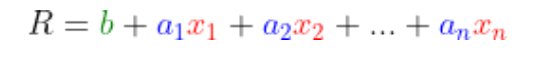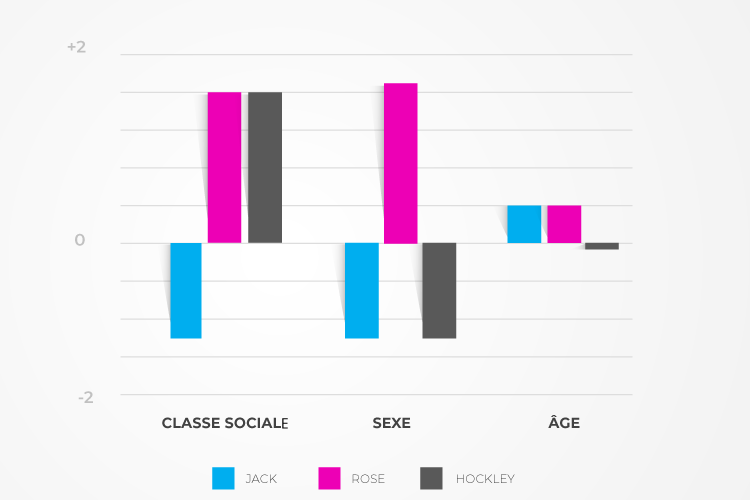Experience shows that this is not enough. Moreover, regulators of financial institutions are not content to know that a model can reduce the default rate on loans, and what they want to know is why a specific decision has been taken.

So how do you get information from a model so that a non-technical user can assess the relevance of the model?

## A simplistic model

### Logistical regression

The simplest solution to provide a simple explanation to a third party is to use a model whose structure is itself easy to explain. Logistics regression is one of the first statistical models used. It is simple to design, easy to optimize and does not require large IT resources.

A quick web search on logistic regression can cause the most allergic to mathematics to think that the announced simplicity of logistic regression is only a decoy.

In fact, behind this ambiguous mathematical expression are extremely accessible concepts. The core of logistic regression is a weighted sum such as the formula below. The x variables are the descriptive variables used in the model.For example, these can be the characteristics of a house in a real estate price prediction model. Variables a and b are the coefficients of the model. This is the value of these variables that we will seek to optimize so that R is as close as possible to the real price of the house, for all the houses we have in our database.

In this formula, R can take any value. In a logistic regression, R is moved into another mathematical formula, the logistic function, to ensure that R is between 0 and 1. To summarize, logistic regression is a weighted sum of descriptive variables, reduced to values between 0 and 1.

### Titanic: an application model

Let’s take as an example the preferred dataset of the apprentice data scientist: the data on the passengers of the Titanic. In this famous tutorial, we try to identify the survivors of this terrible shipwreck. Here we will consider a simple case using simply the social class, sex and age of the passenger. Let’s compare the predictions of our small model for the three protagonists of the famous film of 1999.The hero of the film, Jack, is a third-class man. The model attributes a negative contribution to these characteristics for Jack’s chances of survival. On the other hand, Rose is a first-class woman and thus benefits from the famous adage “Women and children first” (the model is not trained to know that she will jump from the boat to the last second).

Hockley, Rose’s fiancé, does not receive priority to board the rescue boats as a man. However, his first-class passenger status seems to improve his chances. Indeed, several scenes show first-class men in the boats, not just our antagonist.

The model thus shows consistency with our expectations: women and children first, the privileges of the first class, etc. Thus, although the parameters of the model have been optimized apart from any external “expertise”, we can verify that its behaviour is consistent with the expectations of the “experts”. Through this work of studying explicability, we gain confidence in the model.

## A technique to explain them all

When we want to use more complex models, then the direct explanation of the model as we have just done is no longer possible. Many methods were then developed to try to identify the dominant elements of the result. The most popular method today is based on Shapley’s value.

### The Shapley’s Value

In game theory, Shapley’s value allows us to determine how to “justly” distribute the gains of a collective activity among the participants.

The example proposed in this explanatory video is based on a common taxi trip. Three people share a taxi. The journey costs less than the sum of three individual trips. How can we then distribute equitably the “gain” related to the sharing of the taxi? By assigning to each person the fraction of the gain corresponding to its value of Shapley. This video (this time in French) offers another case study.

As soon as a model becomes somewhat complex, the variables can have combined effects. In the previous model, the impact of the class was identical, regardless of the gender of the person. However, one can easily imagine that the difference between man and woman in chances of survival is much more marked in the third class than in the first. It then becomes difficult to estimate the contribution of each variable independently.

Shapley’s value allows us to answer this problem. For a given person, the chances of survival can be calculated if we consider only one subset of the available characteristics.

For example, one can calculate one’s chances without considering the gender or class of the person, and then repeat the operation for all possible subsets.

By making weighted averages of the chances of survival (Shapley method) for each subassembly, one is able to obtain the importance of each of the variables for that specific person.

### Understanding data science techniques by trades as a key to trust

A method (rather popular) has been detailed here. There are many others, sometimes specific to a domain (image analysis…). Here, the details of a technique are less important than the reason for the details.

It has already been mentioned that the confidence of trade experts in AI systems depends in part on explicability. The trade could quite simply be satisfied with the raw explanations provided by a given method.

However, the explanation models only give a biased picture of the reality of the model. For Shapley, for example, the contribution of each variable depends on the characteristics of our anonymous passenger.

It is therefore important that trade experts have knowledge of the models used and their visualization techniques in order to be able to collaborate more and more effectively in the creation of useful models.